•软件开发
• 修改这行代码 换成你的图片所在地方即可A imread "C: Users lenovo Desktop 1 jpg" ;
• 入门matlab图像处理图像的基本操作，包括详细的代码资料和讲解注释
• Matlab 图像处理基本操作 文/天神   一、图像基本操作 1.读取图像并显示： >> clear;close all %清空Matlab工作平台所有变量（准备工作） >> I=imread('pout.tif'); % 该图像Matlab图形工具箱...

Matlab 图像处理基本操作

文/天神

一、图像基本操作

1.读取图像并显示

>> clear;close all         %清空Matlab工作平台所有变量（准备工作）
>> imshow(I)

2.检查内存（数组）中的图像

>> whos

Name      Size                    Bytes   Class

I       291x240                   69840  uint8 array

Grand total is 69840 elements using 69840 bytes

表示该图像采用8位存储方式并占用了69840B的存储空间。

3.实现图像直方图均衡化：

>> figure,imhist(I)    % 在新图中显示图像I的直方图>> I2=histeq(I);  % 均衡化以后的图像存在I2数组中(图像灰度值扩展到整个灰度范围,对比度提高)
>> figure,imshow(I2)  % 显示均衡化后的图像
>> figure,imhist(I2)   % 显示均衡后的灰度值分布情况

8位图像取值范围：[0,255]，16位图像取值范围：[0,655351]，双精度图像取值范围：[0,1]

4.保存图像：

>> imwrite(I2,'pout2.png');    % 将图像由原先的tif格式另存为png格式

5.检查新生成文件的信息：

>> imfinfo('pout2.png')     % 观察保存图像的文件信息

ans =

Filename: 'pout2.png'       % 文件名
FileModDate: '11-Apr-2009 21:55:35'   % 文件修改日期
FileSize: 36938       % 文件大小
Format: 'png'       文件格式
FormatVersion: []          % 格式
Width: 240        % 文件宽度
Height: 291        % 文件高度
BitDepth: 8          % 文件位深度
ColorType: 'grayscale'  % 颜色类型

……

二、图像处理Matlab的应用：

实例：消除rice.png图像中亮度不一致的背景，并使用阈值将修改后的图像转换为二值图像，使用成员标记返回图像中对象的个数以及统计特性。按照如下步骤进行：

1.读取和显示图像

>> clear;close all
>> imshow(I)

2.估计图像背景：

图像中心位置背景亮度强于其他部分亮度，用imopen函数和一个半径为15的圆盘结构元素对输入的图像I进行形态学开操作，去掉那些不完全包括在圆盘中的对象，从而实现对背景亮度的估计。

>> clear;close all
>> imshow(I)
>> background=imopen(I,strel('disk',15));
>> imshow(background)
>> figure,surf(double(background(1:8:end,1:8:end))),zlim([0,255]);
>> set(gca,'ydir','reverse');

显示了背景图（左）和背景表面图（右）

3.从原始图像中减去背景图像（原始图像I减去背景图像得到背景较为一致的图像）：

>> I2=imsubtract(I,background);
>> figure,imshow(I2)

>> figure,imshow(I3);

5.使用阈值操作将图像转换为二进制(二值)图像(bw),调用whos命令查看图像的存储信息.

>> level=graythresh(I3);   % 图像灰度处理
>> bw=im2bw(I3,level);     % 图像二值化处理
>> figure,imshow(bw)       % 显示处理后的图片

>> whos
Name             Size                    Bytes  Class

I              256x256                   65536  uint8 array
I2             256x256                   65536  uint8 array
I3             256x256                   65536  uint8 array
background     256x256                   65536  uint8 array
bw             256x256                   65536  logical array
level            1x1                         8  double array

Grand total is 327681 elements using 327688 bytes

6.检查图像中对象个数（bwlabel函数表示了二值图像中的所有相关成分并返回在图像中找到的对象个数）

>> [labeled,numObjects]=bwlabel(bw,4);

>> numObjects

numObjects =

101

表示图像中的米粒对象个数是101.

7.检查标记矩阵：（imcrop命令进行交互式操作，图像内拉出较小矩形并显示已标记的对象和部分背景内的像素）

>> grain=imcrop(labeled)

grain =

0     0    42    42    42    42    42    42    42     0
0     0    42    42    42    42    42    42    42    42
0     0    42    42    42    42    42    42    42    42
0     0    42    42    42    42    42    42    42    42
0     0    42    42    42    42    42    42    42    42
0     0    42    42    42    42    42    42    42    42
0    42    42    42    42    42    42    42    42    42
0    42    42    42    42    42    42    42    42    42

8.观察标记矩阵（用label2rgb将其显示为一副伪彩色的索引图像）:

>> RGB_label=label2rgb(labeled,@spring,'c','shuffle');
>> imshow(RGB_label);

9.测量图像对象或区域的属性（Regionprops，返回一个结构数据）

>> graindata=regionprops(labeled,'basic')

graindata =

101x1 struct array with fields:
Area
Centroid
BoundingBox
>> graindata(40).Area   % 显示矩阵中第40个元素的属性

ans =

197

>> graindata(40).BoundingBox,graindata(40).Centroid    % 寻找最近的边缘和中心点

ans =

82.5000   59.5000   24.0000   20.0000

ans =

95.4213   70.4924

>> allgrains=[graindata.Area];     % 创建一个新的向量allgrains,其包含每个米粒的范围
>> whos allgrains
Name            Size                    Bytes  Class

allgrains       1x101                     808  double array

Grand total is 101 elements using 808 bytes

>> allgrains(51)     % 相当于整个矩阵的索引为51的属性是多少，可见与原来得到的结果相同

ans =

140

>> max(allgrains)    % 获取最大的米粒大小

ans =

404
>> biggrain=find(allgrains==404)    % 使用find命令返回这个最大尺寸米粒的标记号

biggrain =

59

>> mean(allgrains)   % 获取米粒的平均大小

ans =

175.0396

9.绘制包含30个柱的直方图来说明米粒大小的分布情况

>> hist(allgrains,30)

(米粒大小分布柱状图)

相关链接：

展开全文matlab 图像处理 图片 标准
• MATLAB图像的基本操作及运算

千次阅读 多人点赞 2019-05-08 19:17:35
目录 一、内联函数与匿名函数 （一）内联函数 1. 特点 2. 语法 ...二、MATLAB GPU 编程...三、图像基本操作 （一）数字图像的离散化表示 1.灰度图像 2.RGB 图像 3. 索引图像 （二）图像的读、写与显示 ...

目录

一、内联函数与匿名函数

（一）内联函数

1. 特点

2. 语法

3. 优势

（二）匿名函数

1. 特点

2. 语法

3. 例子

二、MATLAB GPU 编程基础

（一）GPU设备确认

（二）CPU与GPU之间的数据交互

三、图像的基本操作

（一）数字图像的离散化表示

1.灰度图像

2.RGB 图像

3. 索引图像

（二）图像的读、写与显示

（三）图像的邻域操作

1. 定义

2.类型

3.滑动邻域操作

4.图像的块操作

四、图像的基本运算

（一）代数运算

1. 图像的叠加运算

2. 绝对差函数

3.图像减法运算

4.图像乘法运算

5.除法运算

6.图像的线性组合

7.图像求补运算

（二）几何变换

1.改变图像大小

2.图像剪切

3.图像旋转

4.图像的几何形变

一、内联函数与匿名函数

（一）内联函数

1. 特点

在命令窗口、程序或函数中创建局部函数，使用inline function不需要将其存储为一个.m文件，同时又可以像一般函数一样去调用它。

2. 语法

fhandle=inline(expr,arg1,arg2...)

其中，fhandle：函数句柄；expr：函数表达式；argi：函数参数

3. 优势

由于内联函数存储在内存中而不是.m文件中，所以省去了文件访问的时间，加快了程序运行的效率。

（二）匿名函数

1. 特点

用户可以编写简单的函数而不需要创建.m文件。具有inline函数的所有优点，比inline函数的运算效率还要高。

2. 语法

fhandle=@(arglist)expression

fhandle:函数句柄；arglist:参数列表

3. 例子

e.g.使用内联函数和匿名函数定义

f1=inline('x^2+y^2','x','y')
f2=@(x,y)x^2+y^2

（一）GPU设备确认

1. gpuDeviceCount 用于获取当前设备上的GPU的数量，语法格式为：

m=gpuDeviceCount

2.gpuDevice 用于选择GPU设备，语法格式为：

d=gpuDevice
%or
d=gpuDevice()

如果当前还未设置选择的GPU，则选择默认的GPU，D是返回对象；如果已经设置好了GPU，则返回设置的GPU对象。

d=gpuDevice(IDX)

表示选择IDX对应的GPU设置，D为返回对象

3. reset用于清空GPU内存，语法格式为：

reset(gpudev)

与clear命令功能类似，gpudev是gouDevice返回的对象。

4.例子

e.g.获取当前计算机的GPU数目，指定GPU设备，并清空所指定的GPU的内存

n=gpuDeviceCount
D=gpuDevice
reset(D)

（二）CPU与GPU之间的数据交互

1. gpuArray 用于将CPU内存的数据传到GPU内存中，语法格式为：

GX=gpuArray(x)

x为CPU中的内存变量，GX为GPU中的内存变量

2. gather 用于将GPU内存数据传到CPU内存中，语法格式为：

X=gather(GX)

GX为GPU中的内存变量，X为得到的CPU中的内存变量。

3. 例子

e.g.编程实现CPU与GPU之间内存变量的交互

X=rand(10,'single');   %单精度10*10数组（单精度每个数占4个字节）
GX=gpuArray(X);        %在GPU定义变量GX，并将X的值传给GX
GX2=GX.*GX;            %在GPU执行数组对应位置的点乘
X2=gather(GX2);        %将在GPU上的运算结果GX2返回到CPU

e.g.编程比较CPU与GPU对大规模计算的运算效率

%CPU
A1=rand(12000,400);
B1=rand(400,12000);
tic
f1=sum(A1.'.*B1,1);    %A1.':转置，.*对应元素相乘，1:按列相加
tCPU=toc

%GPU
A2=rand(12000,400,'gpuArray');
B2=rand(400,12000,'gpuArray');
tic
f2=sum(A2.'.*B2,1);
tGPU=toc

三、图像的基本操作

（一）数字图像的离散化表示

1.灰度图像

在MATLAB中图像是以二维矩阵的形式来表示的，矩阵中每一个元素代表一个像素，因此该矩阵也称为像素矩阵。元素值越大，对应的像素越亮。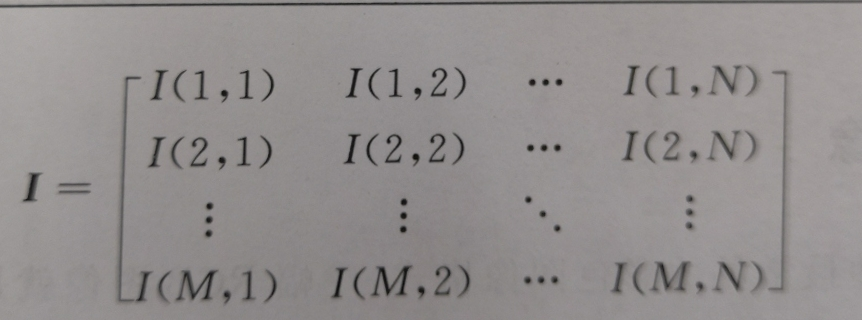在MATLAB中，矩阵元素的下标约定从（1，1） 开始；

2.RGB 图像

一幅分辨率为M✖️N的灰度图像，对应的数值矩阵为M✖️N维的矩阵，各个元素值的范围为[0,255];

一幅分辨率为M✖️N的RGB图像，对应的数值矩阵为M✖️N✖️3，分别对应R、G、B分量。

如果一幅图像的红色分量图比较亮，说明该RGB图像主要呈现为红色。

3. 索引图像

索引图像有两个分量，即整数的数据矩阵X和彩色映射矩阵map；map为m✖️3的浮点类型，m为其所定义的颜色数目，每一行定义了颜色的红、绿、蓝三个分量。如下图，左边数据矩阵存储每个像素点的颜色索引，右边矩阵存储图像所包含的所有颜色的RGB分量值。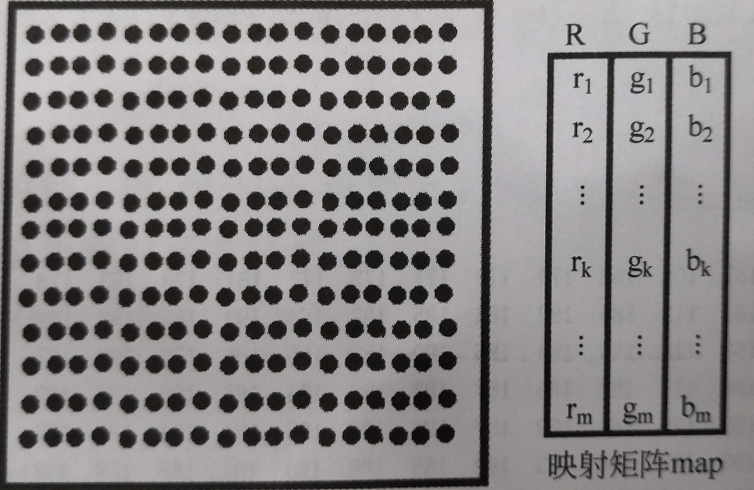（二）图像的读、写与显示

%读取图像
%map为彩色映射矩阵（若读取非索引矩阵，此参数可省略）

%图像大小
[m n]=size(I)

%显示图像
imshow(I)
imshow(I,[low high]);%将<=low的灰度显示为黑色，将>=high的灰度显示为白色
imshow(I,[]) %自动将low设置为I中的最小值，将high设置为I中的最大值;
%当图像矩阵的动态范围较小或有负值出现时经常用到

%保存图像
imwrite(I,filename);  %将图像以文件名filename保存在存储器中，
%filename应包含扩展名，指定文件类型

（三）图像的邻域操作

1. 定义

将每个像素值利用其相应邻域信息进行处理的过程，所选的像素邻域通常远小于原图像的大小，且形状规则。

2.类型

滑动邻域操作和分离邻域操作

3.滑动邻域操作

（1）特点

1. 滑动邻域是一个像素集，所包含的像素由中心像素的位置决定，操作的结果也作为该中心像素新的灰度值；
2. 滑动邻域操作一个邻域只处理一个像素，即中心像素；为方便确定中心像素，通常采用奇数行、奇数列的矩阵窗口。

（2）实现步骤

1. 选择一个单独的像素作为中心像素；
2. 确定该像素的滑动邻域；
3. 对邻域中的像素值应用一个函数求值，该函数将返回标量计算结果；
4. 将计算结果作为输出图像中对应的像素点的值；
5. 对输入图像的每个像素都重复上面4个步骤。

（3）函数实现

J=nlfilter(I,[m n],fun)
J=nlfilter(I,'indexed',[m n],fun)

其中，I为原图像；[m n]为指定大小为m✖️n的滑动邻域；

fun是对滑动邻域矩阵进行操作的函数，fun可以为函数句柄，用户可以定义匿名函数，提高代码效率

indexed是可选参数，指定这个参数，则表示原图像为索引图像。

e.g.使用nlfilter实现将每个像素设置为5✖️5邻域的最大值。

%myf=@(I)max(I(:));
%y=nlfilter(I,[5,5],myf);
y=nlfilter(I,[5,5],@my_max);
subplot(121);imshow(I);title('原图')
subplot(122);imshow(y);title('邻域操作结果')
figure
y=nlfilter(I,[5,5],@my_median);
subplot(121);imshow(I);title('原图')
subplot(122);imshow(y);title('邻域操作结果')

function y=my_max(x)
y=max(x(:));
end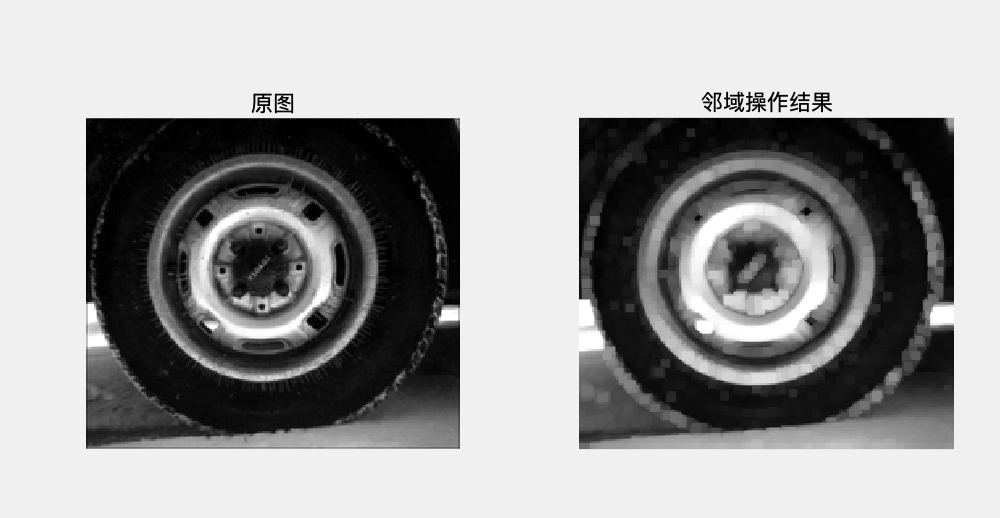(4)快速邻域块操作

colfilt函数为每个像素建立一个列向量，向量各元素对应该像素的邻域元素，减少了图像操作过程中的邻域定义的耗时。

J=colfilt(I,[m n],block_type,fun)
J=colfilt(I,[m n],[mblock unblock],block_type,fun)
J=colfilt(I,'indexed',...)

其中，I表示原图像；[m n]为指定大小为m✖️n的邻域块；

[mblock nblock]表示一次将大小为mblock✖️nblock大小的图像读入内存；

fun为邻域矩阵操作函数；indexed用于指明索引图像的可选参数；

block_type用于定义块的移动方式，'distinct'表示分离块操作，'sliding'表示滑动块操作。

e.g.使用colfilt实现快速滑动邻域操作

myf=@(I)max(I);
y=colfilt(I,[5 5],'sliding',myf);
subplot(121);imshow(I);title('原图')
subplot(122);imshow(y);title('邻域操作结果')

由于colfilt函数操作中，每个像素的邻域已排成列向量，所以这里的匿名函数为max(I)不同于nlfilt的max(I(:))。

（即在colfilt中，[m n]大小的邻域矩阵已排成列向量，fun编写时输入为列向量；而nlfilt中[m n]仍未二维矩阵形式，fun编写时其输入为二维矩阵。）

4.图像的块操作

图像的分离块操作是将图像划分为大小相同的矩形区域，不同图像块在图像中无重叠排列。

J=blockproc(I,blockSize,fun)

其中，I为原图像；

blockSize用于指定图像块的大小，图像块的大小由块结构定义，相应的数据矩阵放在.data域中。

e.g.将32✖️32的图像块中所有像素的灰度值设为该图像块的标准方差。

%fun=@(X)std2(X.data)*ones(size(X.data));
I2=blockproc(I,[32 32],@std);
subplot(121);
imshow('moon.tif');title('原图');
subplot(122);
imshow(I2,[]);title('块操作后图像');

function y=std(x)
y=std2(x.data)*ones(size(x.data));
end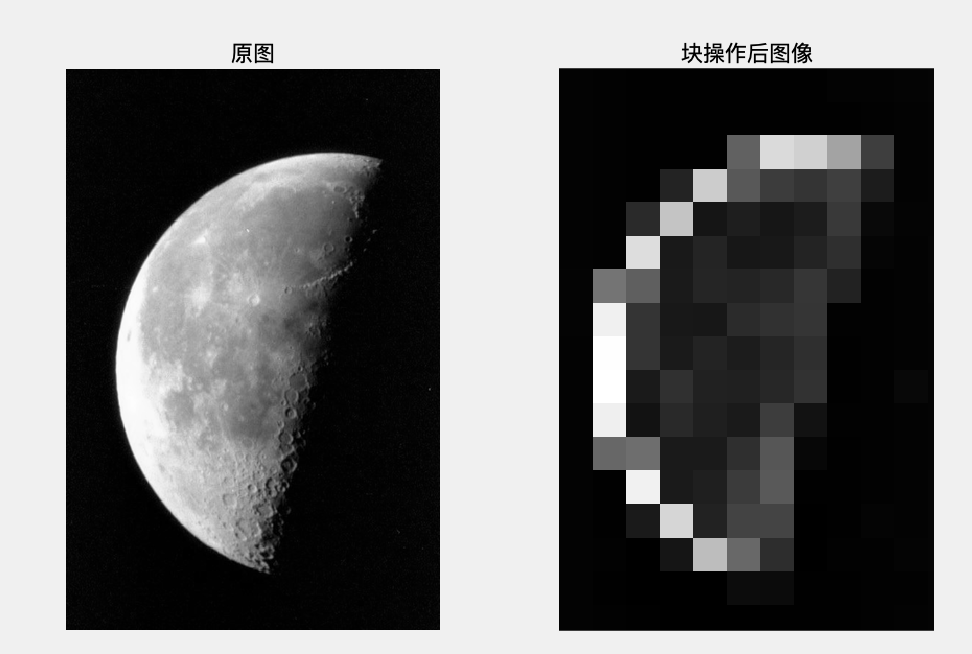四、图像的基本运算

（一）代数运算

• 图像的代数运算又称图像的算术运算，指多幅图像或多个波段图像间做相应像素的逐点加、减、乘、除等运算。
• 可以直接使用MATLAB运算符对图像矩阵进行操作，但需先将图像矩阵转换为与数值矩阵运算相一致的double类型，运算结束后还应转换回图像原数据类型。

1. 图像的叠加运算

其中，X、Y为两个大小相同的图像矩阵。

e.g. 1 对MATLAB工具箱中rice.png和cameraman.tif图像进行叠加操作

subplot(131);
imshow(X);xlabel('(a)');
subplot(132)
imshow(Y);xlabel('(b)');
subplot(133)
imshow(Z);xlabel('(c)');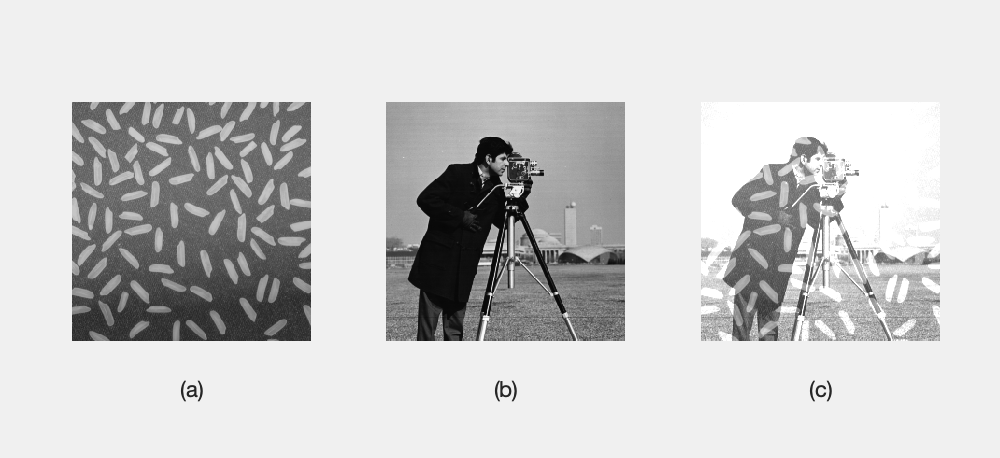subplot(121);
imshow(I);title('原图')
subplot(122);
imshow(J);title('提亮后')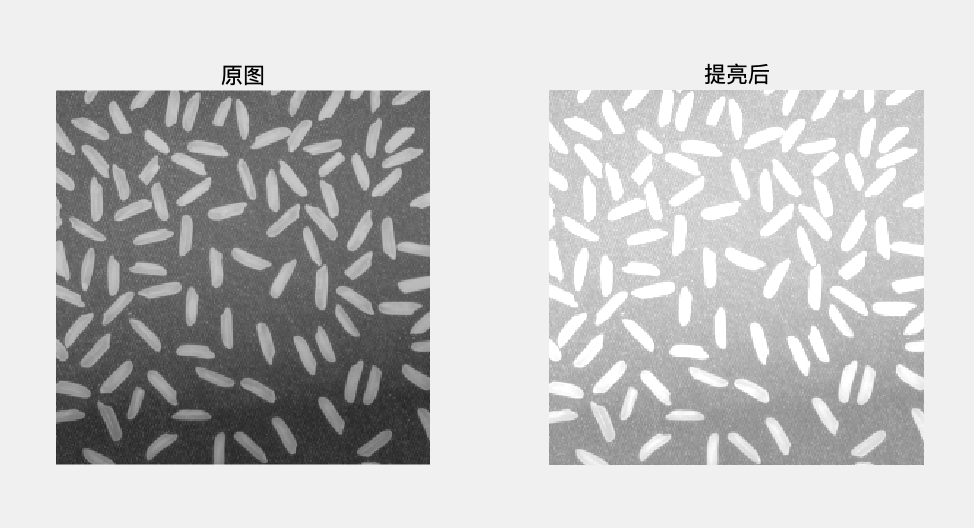2. 绝对差函数

对图像进行绝对差处理可以显示一张经过邻域处理的图像与原图的差别。

Z=imabsdiff(X,Y)

其中，X、Y为大小相同的两个图像矩阵；

Z为X、Y两个矩阵分别做减法并对每一个元素分别取相应差的绝对值的结果矩阵。

e.g.显示经过邻域处理的图与原图的差别

myf=@(x)mean(x);
y=uint8(colfilt(x,[7,7],'sliding',myf));
z=imabsdiff(x,y);
subplot(131);imshow(x);title('原图')
subplot(132);imshow(y);title('邻域处理')
subplot(133);imshow(z);title('绝对差值图像')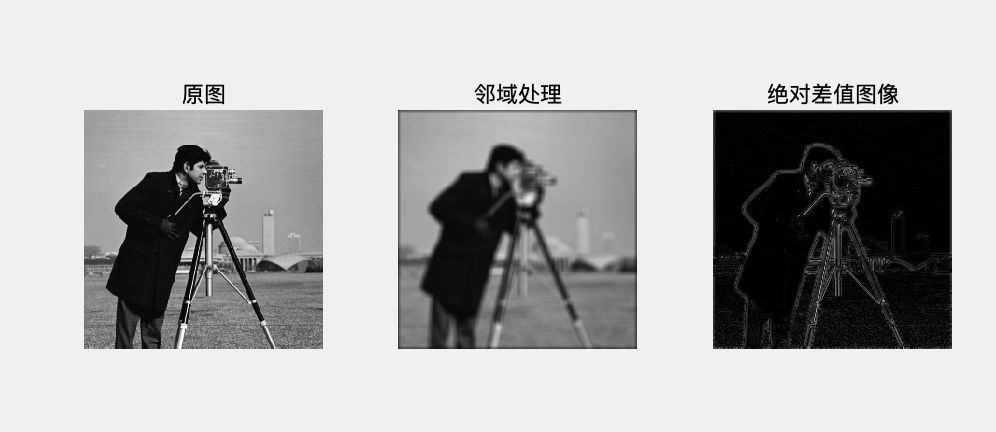3.图像减法运算

图像减法运算通常用于检测图像变化，例如视频中两相邻帧之间的变化。

Z=imsubtract(X,Y)

其中，X、Y为大小相同的两个图像矩阵；

Z为X、Y两个矩阵各个元素做减法，当差小于0时，直接将结果设为0。

4.图像乘法运算

immultiply函数可将两幅图像相乘或将一幅图像与一个常数相乘。常用于掩膜操作（去掉图像中某些部分）或图像灰度增强。

Z=immultiply(X,Y)

5.除法运算

Z=imdivide(X,Y)
1. 操作对象可以是两幅图像，也可以是一幅图像与一个常数；
2. 运算结果是像素值的相对变化比率，而非绝对差异。（这是与图像相减的不同。）

e.g. 应用实例

imshow(z,[]);%显示动态范围小的图片

myf=@(x)(min(min(x.data)))*uint8(ones(size(x.data)));
y=blockproc(x,[15 15],myf);
z=imdivide(x,y);
s=imdivide(x,2);

subplot(221)
imshow(x);title('原图')
subplot(222)
imshow(y);title('邻域处理后')
subplot(223)
imshow(z,[])     %z矩阵动态范围小
title('相除')
subplot(224)
imshow(s);title('与常数相除')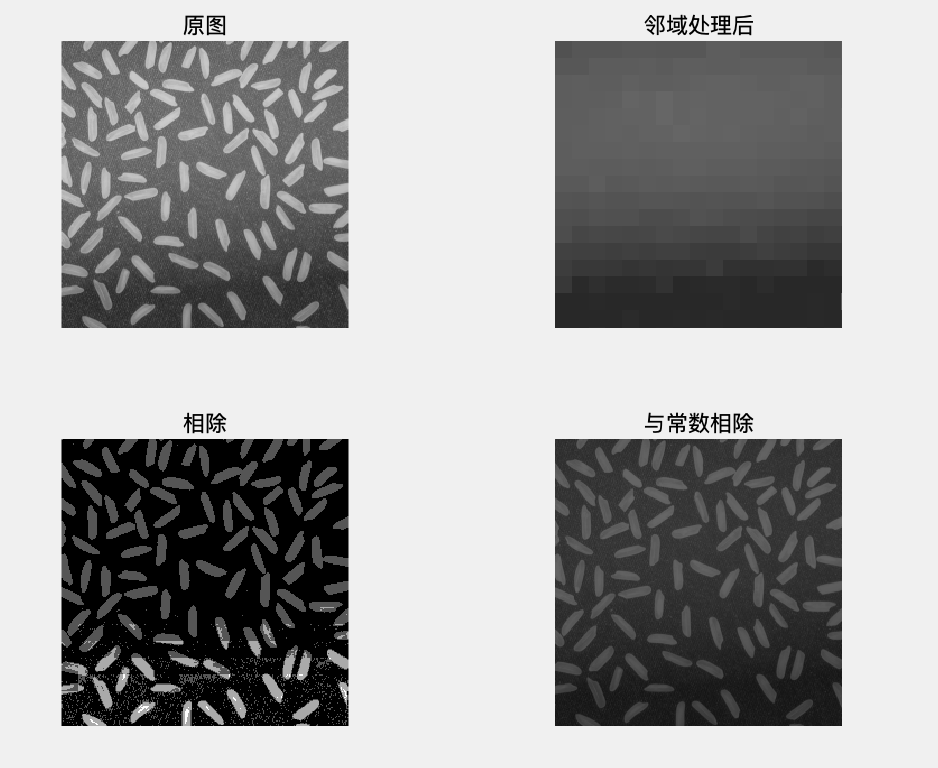6.图像的线性组合

Z=imlincomb(K1,A1,K2,A2,...,Kn,An)
Z=imlincomb(K1,A1,K2,A2,...,Kn,An,K)
Z=imlincomb(...,output_class)

其中，Ki为双精度浮点标量；Ai为图像矩阵；K为常数项；output_class用来指定输出变量的数据类型。

（将Ai读入GPU后，可在GPU上运行。）

e.g.使用imlincomb实现图像的线性组合。

K=imlincomb(1,I,3,J,'uint16');
subplot(131)
imshow(I);xlabel('a');
subplot(132)
imshow(J);xlabel('b');
subplot(133)
imshow(K,[]);xlabel('c');

%GPU实现
K=imlincomb(1,I,3,J,'uint16');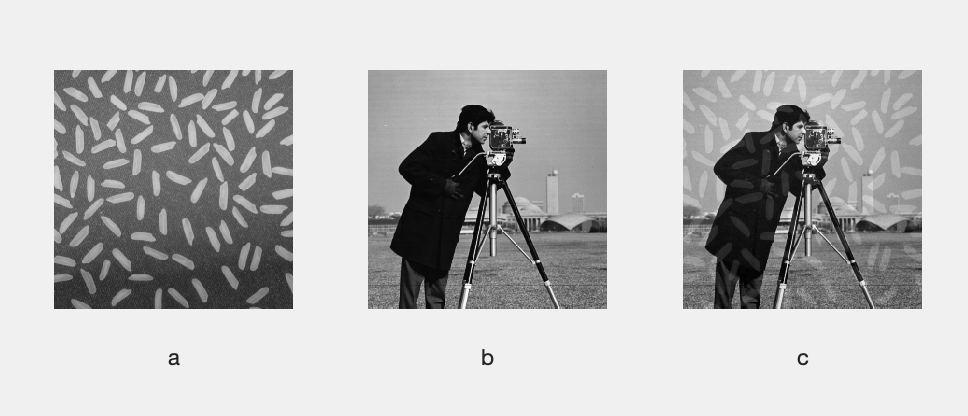7.图像求补运算

IM2=imcomplement(IM)

其中，IM可以为二值图像、灰度图像或RGB图像；输出的补图IM2与IM具有相同的图像大小和图像类型。

若为灰度图像，IM2各个元素的值为（255-IM各个元素的值）。

（二）几何变换

• 对图像进行几何变换时，像素坐标将发生改变，需进行插值操作，即利用已知位置的像素值生成未知位置的像素点的像素值。
• 常见的插值方法有：最近邻插值（'nearest')，线性插值（'linear'），三次插值（'cublic')，双线性插值（'bilinear'）,双三次插值（'bicubic'）。

1.改变图像大小

保持图像形状的基础上对图像的大小进行改变。

B=imresize(A,scale,method)
B=imresize(A,outputSize,method)
[Y,newmap]=imresize(X,map,...)

其中，A为原图；scale是进行大小改变的倍数；method为可选项，指定插值方法，可选'nearest','bilinear','bicubic'，默认为‘bicubic'

outputSize为输出图像的行和列的维数，通常以[numrows numcols]的方式给出;

X为索引图像，map为对应的映射表；Y为输出索引图像，newmap为Y的映射表。

2.图像剪切

I2=imcrop   %未指定原图像，把当前图形窗口中的图像作为待剪切的图像
I2=imcrop(I)
X2=imcrop(X,map） %处理索引图像
I3=imcrop(h)       %h为图像句柄

I2=imcrop(I,rect)    %rect指定剪切区域的坐标及大小，分别为X，Y方向的最小值及矩形区域的宽与高
X2=imcrop(X,map,rect)

[I2,rect2]=imcrop(I,...)   %输出剪切结果的同时还输出此剪切图像在原图中的位置

3.图像旋转

I2=imrotate(I,angle,method,bbox)

其中，angle为旋转角度；method为插值方法，可选'nearest','bilinear','bicubic'，默认为'nearest'；

bbox为旋转后图像边界选项，有'crop'和‘loose’（默认）两个选项，前者通过剪切图像，使旋转先后图像大小一致；后者允许I2包含I的全部信息。

e.g.两种图像边界对比。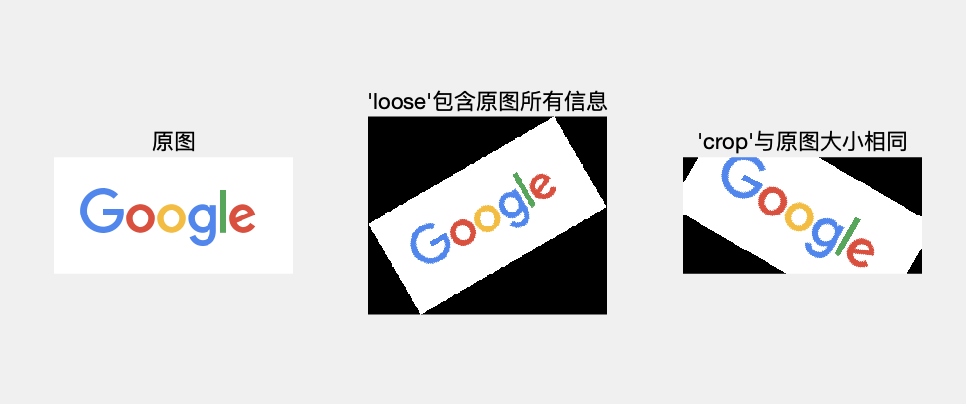4.图像的几何形变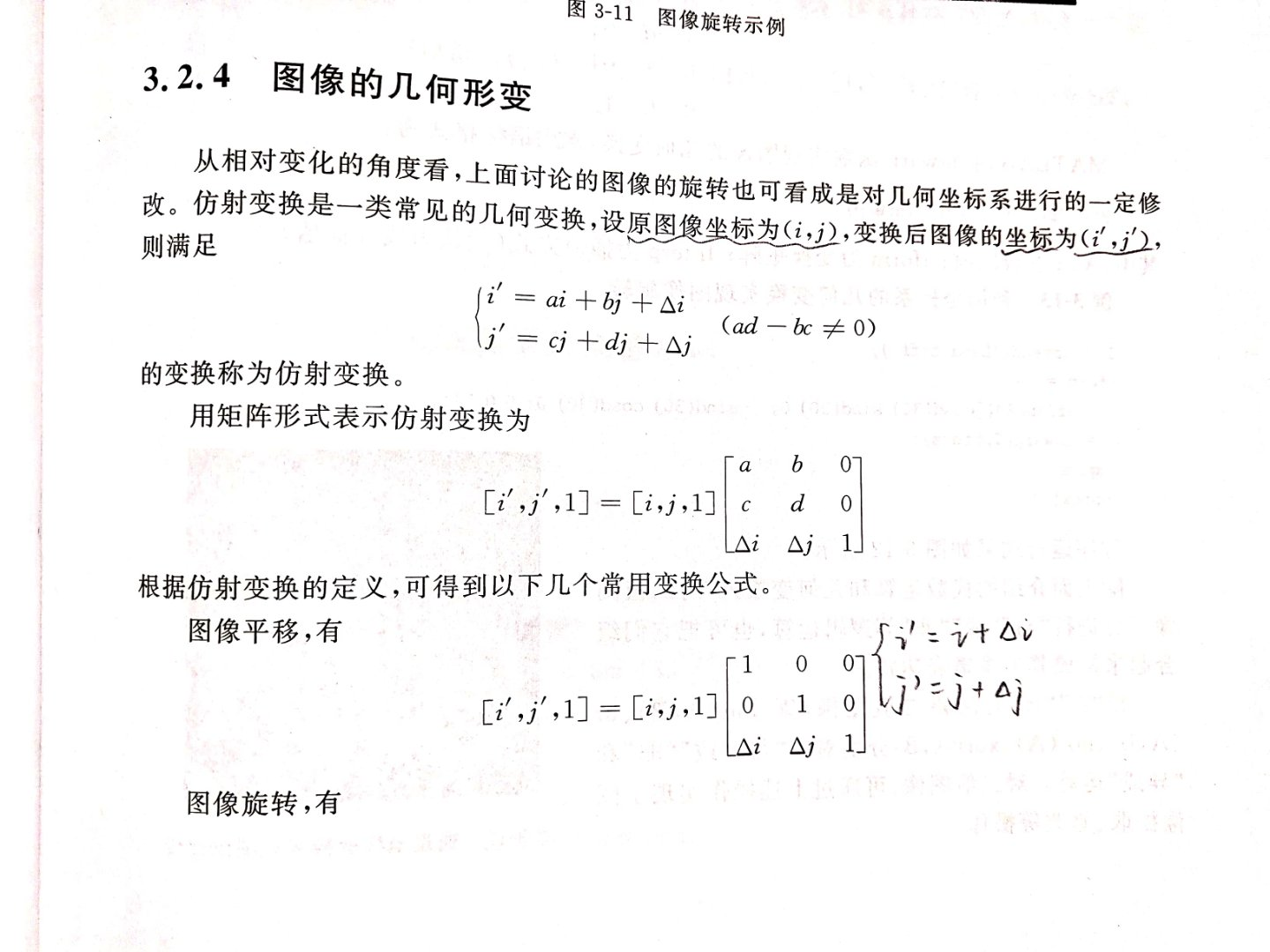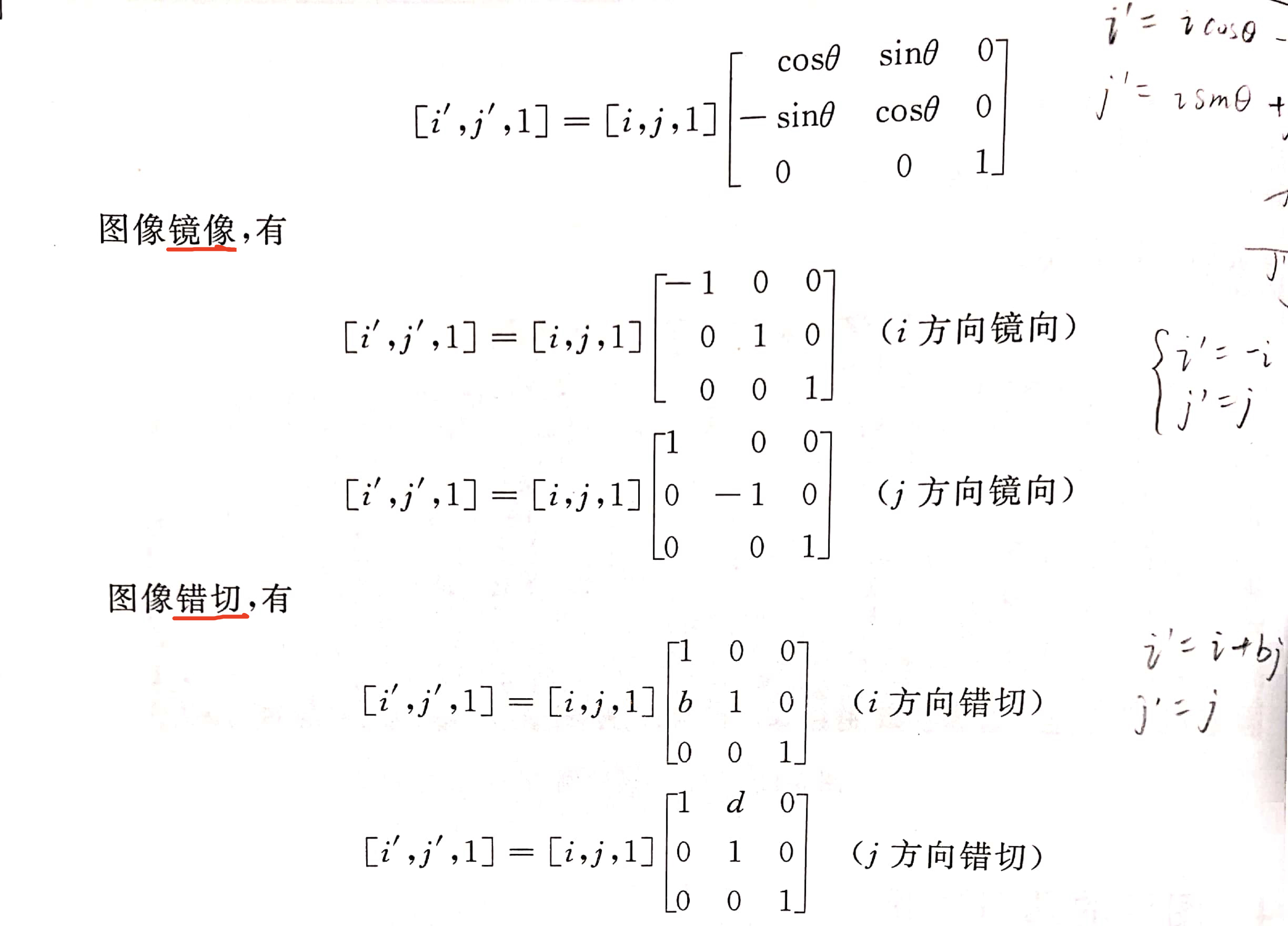I2=imwarp(I,tform,Interp)

其中，tform为变换矩阵；Interp为插值方式（默认为线性插值）。

e.g.利用坐标系的几何变换实现图像旋转

subplot(121)
imshow(I);title('原图')
tform=affine2d([cosd(30) sind(30) 0;-sind(30) cosd(30) 0;0 0 1]);
J=imwarp(I,tform);
%效果等价于
%J=imrotate(I,-30);
subplot(122)
imshow(J);title('顺时针旋转30度');展开全文• matlab图像的基本操作

千次阅读 2016-08-17 13:00:52
（1）显示：image()可以直接显示8位数图像，但8位图像和double型数据在image（）中意义是不一样的。二值图可用uint8或double来储存； 索引图像uint8和double型显示方法没区别。但8位数据矩阵的值和颜色种类之间有一...

（1）显示：

image()可以直接显示8位数图像，但8位图像和double型数据在image（）中意义是不一样的。

二值图可用uint8或double来储存；

索引图像uint8和double型显示方法没区别。但8位数据矩阵的值和颜色种类之间有一个数值为1的偏差。调用格式 image(x); colormap(map);

对于灰度图像，uint8范围0~255，double范围0~1，二者转换方式： i8 = uint(round(i64*255)); i64 = double(i8)/255 ;

imshow(f,[low,high]) 所有小于low显示黑色，所有大于high显示白色；

imshow(f,G) g表示灰度级数；

imshow(f,[]) 解决uint8范围0~255转换为0~1；

（2）读取：

matlab读入图像是uint8类型，而在矩阵中使用的是double，所以需要转换。im2double()，im2uint8()，im2uint16();

（3）存取：

matlab默认将图片存储double型；也支持uint8或uint16，优势在于节省空间。

mat2gray（）把double型数组转换到0~1之间，将矩阵转换成灰度图像得出double格式。

isgray（）函数判断矩阵是否为图像数据矩阵。

（4）转换：

gray2ind() ind2gray() [x,map]=rgb2ind() ind2rgb(x,map) rgb2gray() im2bw(rgb,level)阈值

（5）写入：

imwrite(I,’snight.jpg’) 或imwrite(I,’snight’,’jpg’)

展开全文matlab
• %% 学习目标：学习关于图像基本操作 %% 通过抖动来增强图像的的色彩对比度 clear all; close all; I = imread('cameraman.tif');%读取灰度图像 BW = dither(I);%通过抖动转换为二值图像来增强图像的色彩对比度 ...
%% 学习目标：学习关于图像的基本操作

%% 通过抖动来增强图像的的色彩对比度
clear all;
close all;
BW = dither(I);%通过抖动转换为二值图像来增强图像的色彩对比度
subplot(1,2,1);%将多个图片显示在同一个窗口，subplot(m,n,p)。
imshow(I);
subplot(1,2,2);
imshow(BW);

%% 获取图像信息
clear all;
close all;
info = imfinfo('trees.tif','tif');%获取图像信息
disp(info);%输出图像信息

%% 改变图像格式
clear all;
close all;
imwrite(RGB,'Mytrees.png','png');%将tif格式图片转换为png格式
figure;
imshow(tupian);

%% 读取图像中的某些帧
clear all;
close all;
figure;
subplot(1,2,1);
imshow(I1);
subplot(1,2,2);
imshow(I20);

%% which mri.tif 查看图像位置

%% 同时显示多帧图像
clear all;
close all;
mri = uint8(zeros(128,128,1,25));
for i = 1:25  %25帧
end
montage(mri,map);%同时显示多帧图像

%% 将多帧图像转换为电影
clear all;
close all;
mri = uint8(zeros(128,128,1,25));
for i = 1 : 25
end
mov = immovie(mri,map);%把图像转换为动画
implay(mov);%显示动画

%% 图像相加  如果大于255就设置为255
clear all;
close all;
imshow(K,[]);

%% 图像相减，小于0则设置为0，  0到255  减去一个常数颜色更深
clear all;
close all;
J = imsubtract(I,90);%减去90  减的越大，图像越暗（0表黑色嘛）
imshow(J);

%% 图像相乘
clear all;
close all;
J = immultiply(I,0.6);%乘0.6（小于1），变暗
subplot(121);
imshow(I);
subplot(122);
imshow(J);

%% 图像相除  维数要一样
clear all;
close all;
X = uint8([222 50 21; 56 77 89]);%X and Y 都是2*3的矩阵
Y = uint8([66 66 66; 66 66 66]);
Z = imdivide(X,Y);% X/Y
disp(Z);

%% 两幅图像的绝对差异
clear all;
close all;
J = uint8(filter2(fspecial('gaussian'),I)); % 对图像进行滤波
K = imabsdiff(I,J);%获取滤波图像和之前图像的差异
subplot(121);
imshow(I);
subplot(122);
imshow(K,[]);%加[]是为了显示清晰

%% fspecial：用于建立预定义的滤波算子或者说产生预定义滤波器

展开全文• MATLAB图像处理基本操作(1)

千次阅读 2020-10-03 22:51:38matlab 图像基本操作
• Matlab图像处理，输入与输出部分课程讲义，内含代码
• %提高图像对比度 imhist();%展示直方图 im2bw(I,level);%二值化图像 level为阈值 Q = cumtrapz(Y) ；%计算 Y 的近似累积积分。 imrotate(I,angele);%将图像旋转一定的角度 imresize(I,SIZE);%放大或缩小一定的倍数 %...
• 包括很多相关实验图像处理的相关matlab程序，包括图像处理的基本函数的使用示例，还有很多图像处理经典案例分享，程序操作性强，适合初学者使用matlab
• MATLAB图像操作

2017-09-25 14:16:35
MATLAB操作和使用方法的PPT，其中包括MATLAB的安装，使用方法，MATLAB中的主要函数的使用方法，MATLAB函数,MATLAB基本运算等有关MATLAB软件的操作和使用方法MATLABMATLAB
• 虽然说Matlab是一款数学处理软件，但是这款软件特别强大，强大之处在于他还能够处理图像，这个功能是很多新手学习Matlab的时候感到惊讶的地方，所不了解，所要学习的，下面小编就给大家介绍Matlab处理图像的方法。...
• MATLAB基本操作及概念

千次阅读 2021-01-28 17:23:41
二、MATLAB基本操作 1.系统界面操作的介绍 2.常见操作命令及快捷键 编辑器窗口（Editor）下的常用快捷键 代码调试常用快捷键 命令窗口（Command Window）下的常用快捷键与命令 切换窗口快捷键 3.MATLAB的帮助...编程语言 MATLAB MATLAB基本操作 MATLAB概念 数学建模
• MATLAB数字图像处理基本操作及空域滤波 实验一 MATLAB 数字图像处理基本操作及空域滤波实验目的1、了解有关数字图像处理的基本概念，熟悉Matlab软件中关于数字图像处理的基本命令，掌握利用Matlab软件进行数字图像...matlab空域滤波
• 执行两个图像的加法和减法，低对比度图像的对比度拉伸并找到图像的补码。matlab
• imread : 读取图像 imwrite : 存写图像 imresize ： resize 图像 horzcat, vertcat ： 拼接图像 imshow： 显示图像 rgb2gray : 彩图转灰度图 灰度图转彩图 imread : 读取图像 *： 如果需要更多信息，如...
• matlab基本操作

2014-09-22 12:38:25
很详细的matlab操作基本教程，从开始的软件操作应用，到数组操作图像处理、统计工具、混合编程等。关键是言简意赅有脉络清晰！是新手上路的“必备良药”。
• 图像处理基本操作一、实验目的二、实验题目三、实验内容3.1 相关知识3.2 实验代码3.3 实验结果四、实验心得 一、实验目的 二、实验题目 三、实验内容 3.1 相关知识 3.2 实验代码 3.3 实验结果 四、实验心得 ...
• 感觉matlab语言和python语言很多类似操作，所以敲起代码来有种“春风得意马蹄疾”的感觉，废话不多说，上代码。下面代码matlab入门没啥问题…算法下载地址如下(如果不需要可以忽略下载，用matlab中自带的算法)：...
• MATLAB数字图像处理基本操作及空域滤波实验一 MATLAB数字图像处理基本操作及空域滤波实验目的1、了解有关数字图像处理的基本概念，熟悉Matlab软件中关于数字图像处理的基本命令，掌握利用Matlab软件进行数字图像处理...
• 实验目的l 掌握MATLAB中数字图像的存储，读取和显示l 掌握MATLAB中的基本数据，图像类型，以及类型之间的互相转换l 掌握数字图像数据的索引和赋值方法l 熟悉并掌握基本的M函数编程知识2.2实验原理2.2.1MATLAB中数字...
• matlab图像处理基本操作

千次阅读 2019-05-15 19:42:10
@matab图像处理基本操作 利用imread( )函数读取一幅图像，假设其名为flower.tif，存入一个数组中； 2．利用whos 命令提取该读入图像flower.tif的基本信息； 3．利用imshow()函数来显示这幅图像； 4．利用imfinfo函数......

matlab图像的基本操作matlab 订阅